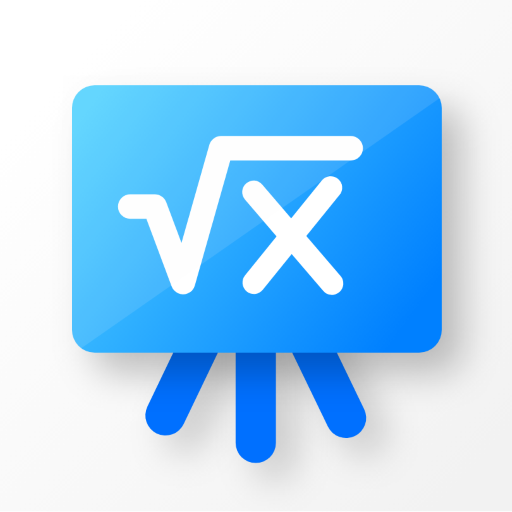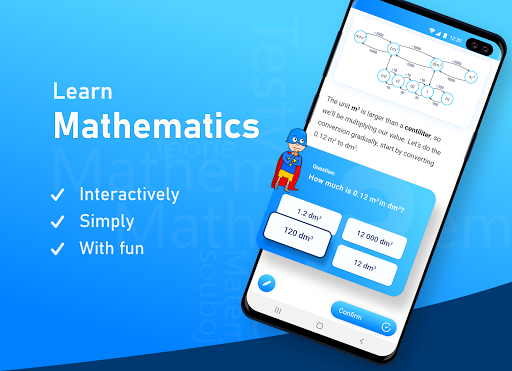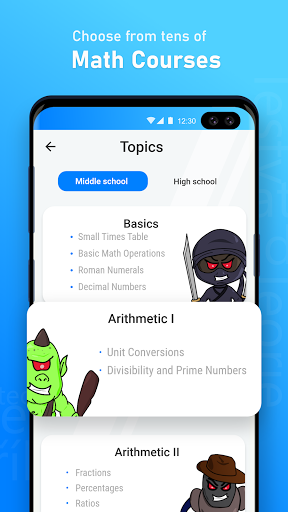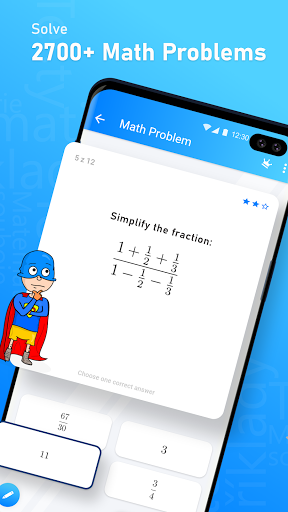# Mathman: Learn Math & Become Math Superhero on PC/Mac

Author: MathLearner

Score: 4.3

OS: Windows, OS X, 5.0 +##### About Mathman: Learn Math & Become Math Superhero
Are you a math learner? 😎 Wanna extra math practice? Are you affraid of math tests? Mathman can help you!

Mathman is the best math practice game to train your brain. Specially designed theory lessons are prepared for you. Learn anything from addition and multiplication, through powers and roots to algebra and complex numbers. Prepare yourself for many standardized test like ACT, GED, SAT, GRE, LSAT, K-12 and others.

But don’t expect a boring textbook. Mathman is a fun math game, where you will fight math monsters, learn math on interactive theory lessons and worksheets and train your brain on hard math problems. Learning math was never easier.

Mathman is built on four pillars.

#### 1) Learn math on interactive theory lessons

In step-by-step theory lessons you’ll learn how to solve math problems like addition, multiplication, powers and roots, algebra, word problems and many more. You won’t just be passively watching! In between steps, you’ll have to answer questions and soon the math theory will seem like second nature.

#### 2) Practice math on 2500+ math problems

Do you wanna some xtra math problems to practice on? No problem. Train your brain on more than 2500 math problems including word problems, long multiplication, fractions, ratios, equations, polynomials, complex numbers and many more.

#### 3) Take a math quiz and defeat math monster

The world needs xtra math heroes, become one today! Each mathematical topic is guarded by a monster. Defeat them to show you’ve mastered the topic.

#### 4) Create your own mathematical tests

Did your math teacher plan a test for you? Don’t worry. With Mathman, you can create your own math quizzes from topics you want. Practice, practice and practice and show your math teacher that you are xtra brilliant math learner.

We’re working on our app day and night, constantly updating it with new math topics. If you’d like to request a math topic to add, let us know at:

Email – info@mathman.cz

Currently available topics include:

☆ Basic Mathematical Operations
☆ Priority of Mathematical Operations

☆ Prime Factorization
☆ Greatest Common Divisor
☆ Least Common Multiple

☆ Counting with Decimal Numbers

☆ Basic Fraction Operations
☆ Reducing Fractions
☆ Complex and Combined Fractions

☆ Counting with Percentages and Ratios

☆ Word Problems for Cross Multiplication
☆ Common Work Problems

☆ Explaining Powers and Roots
☆ Basic Rules for Powers and Roots

☆ Value and Domain of Expressions
☆ Basic Polynomial Operations
☆ Dividing Polynomials by Monomials
☆ Dividing Polynomials by Polynomials
☆ Formulas for Factoring Polynomials
☆ Polynomial Factorization
☆ Rational Expressions

☆ Linear Equations
☆ Word Problems with Linear Equations
☆ Expressing Unknowns From Formulas
☆ Systems of Linear Equations

☆ Solving All Types of Quadratic Equations
☆ Vieta’s Formulas
☆ Standard and Factored Form of Quadratic Equations
☆ Completing the Square

☆ Complex numbers
☆ Nth Powers of Complex numbers
☆ Complex equations

Plus every fortnight we add a new math topic!